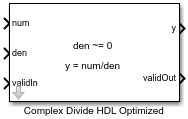Complex Divide HDL Optimized

Divide one input by another and generate optimized HDL code

• Library:
• Fixed-Point Designer HDL Support / Math Operations

•Description

The Complex Divide HDL Optimized block outputs the result of dividing the scalar num by the scalar den, such that y = num/den.

Limitations

Data type override is not supported for the Complex Divide HDL Optimized block.

Ports

Input

expand all

Numerator, specified as a scalar.

Data Types: single | double | fixed point
Complex Number Support: Yes

Denominator, specified as a scalar.

Data Types: single | double | fixed point
Complex Number Support: Yes

Whether input is valid, specified as a Boolean scalar. This control signal indicates when the data from the num and den input ports are valid. When this value is 1 (true), the block captures the values at the input ports num and den. When this value is 0 (false), the block ignores the input samples.

Data Types: Boolean

Output

expand all

Output computed by dividing num by den, such that y = num/den, returned as a complex scalar with data type specified by Output datatype.

Data Types: single | double | fixed point

Whether the output data is valid, returned as a Boolean scalar. When the value of this control signal is 1 (true), the block has successfully computed the output at port y. When this value is 0 (false), the output data is not valid.

Data Types: Boolean

Parameters

expand all

Data type of output y, specified as fixdt(1,18,10), single, fixdt(1,16,0), or as a user-specified data type expression. The type can be specified directly or expressed as a data type object, such as Simulink.NumericType.

Programmatic Use

 Block Parameter: OutputType Type: character vector Values: 'fixdt(1,18,10)' | 'single' | 'fixdt(1,16,0)' | '' Default: 'fixdt(1,18,10)'

Tips

The blocks Divide by Constant HDL Optimized, Real Divide HDL Optimized, and Complex Divide HDL Optimized all perform the division operation and generate optimized HDL code.

• Real Divide HDL Optimized and Complex Divide HDL Optimized are based on a CORIDC algorithm. These blocks accept a wide variety of inputs, but will result in greater latency.

• Divide by Constant HDL Optimized accepts only real inputs and a constant divisor. Use of this block consumes DSP slices, but will complete the division operation in fewer cycles and at a higher clock rate.

expand all

Extended Capabilities

Introduced in R2021a

Fixed-Point Designer DocumentationGet trial now